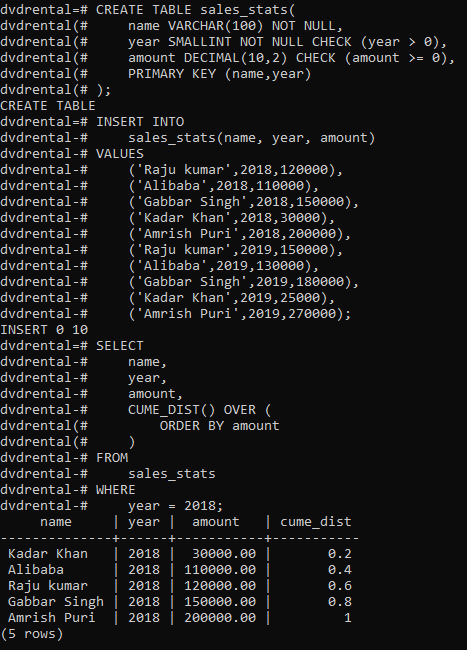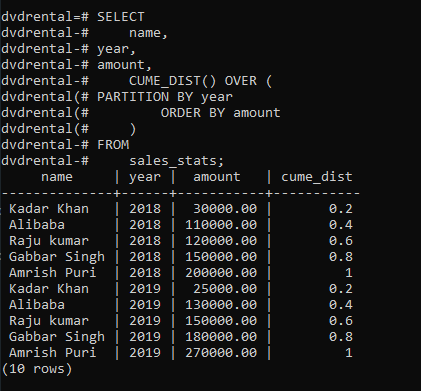# PostgreSQL – CUME_DIST Function

• Last Updated : 10 Feb, 2021

In PostgreSQL, the CUME_DIST() function is used to query for the relative position os a value within a set of given values.

```Syntax:
CUME_DIST() OVER (
[PARTITION BY partition_expression, ... ]
ORDER BY sorting_expression [ASC | DESC], ...
)
```

Let’s analyze the above syntax:

• The PARTITION BY is an optional clause that divides rows into multiple partitions where the function is applied.If not set, postgreSQL treats the whole result set as a single partition.
• The ORDER BY clause sorts rows in each partition where the function is applied.

#### Return Value:

The CUME_DIST() function returns a double-precision value between 0 and 1:

```0 < CUME_DIST() <= 1
```

Example 1:

First, create a table named sales_stats that stores the  sales revenue by employees:

```CREATE TABLE sales_stats(
name VARCHAR(100) NOT NULL,
year SMALLINT NOT NULL CHECK (year > 0),
amount DECIMAL(10, 2) CHECK (amount >= 0),
PRIMARY KEY (name, year)
);
```

Second, insert some rows into the sales_stats table:

```INSERT INTO
sales_stats(name, year, amount)
VALUES
('Raju kumar', 2018, 120000),
('Alibaba', 2018, 110000),
('Gabbar Singh', 2018, 150000),
('Amrish Puri', 2018, 200000),
('Raju kumar', 2019, 150000),
('Alibaba', 2019, 130000),
('Gabbar Singh', 2019, 180000),
('Amrish Puri', 2019, 270000);
```

The following query returns the sales amount percentile for each sales employee in 2018:

```SELECT
name,
year,
amount,
CUME_DIST() OVER (
ORDER BY amount
)
FROM
sales_stats
WHERE
year = 2018;
```

Output:Example 2:

The following query uses the CUME_DIST() function to calculate the sales percentile for each sales employee in 2018 and 2019:

```SELECT
name,
year,
amount,
CUME_DIST() OVER (
PARTITION BY year
ORDER BY amount
)
FROM
sales_stats;
```

Output:My Personal Notes arrow_drop_up